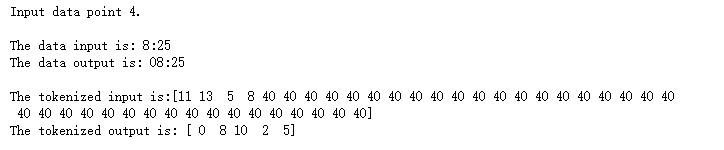• 问答
• 技术
• 实践
• 资源
Python 实现注意力机制

## 引言## 模型实验

### 2.1 数据处理

with open('data/Time Dataset.json','r') as f:    dataset = json.loads(f.read())with open('data/Time Vocabs.json','r') as f:    human_vocab, machine_vocab = json.loads(f.read())human_vocab_size = len(human_vocab)machine_vocab_size = len(machine_vocab)m = len(dataset)def preprocess_data(dataset, human_vocab, machine_vocab, Tx, Ty):    m = len(dataset)    X = np.zeros([m, Tx], dtype='int32')    Y = np.zeros([m, Ty], dtype='int32')    for i in range(m):        data = dataset[i]        X[i] = np.array(tokenize(data, human_vocab, Tx))        Y[i] = np.array(tokenize(data, machine_vocab, Ty))    Xoh = oh_2d(X, len(human_vocab))    Yoh = oh_2d(Y, len(machine_vocab))    return (X, Y, Xoh, Yoh)### 2.2 网络模型设置

layer3 = Dense(machine_vocab_size, activation=softmax)def get_model(Tx, Ty, layer1_size, layer2_size, x_vocab_size, y_vocab_size):    X = Input(shape=(Tx, x_vocab_size))    a1 = Bidirectional(LSTM(layer1_size, return_sequences=True), merge_mode='concat')(X)    a2 = attention_layer(a1, layer2_size, Ty)    a3 = [layer3(timestep) for timestep in a2]    model = Model(inputs=[X], outputs=a3)    return model### 2.3 注意力网络def one_step_of_attention(h_prev, a):    h_repeat = at_repeat(h_prev)    i = at_concatenate([a, h_repeat])    i = at_dense1(i)    i = at_dense2(i)    attention = at_softmax(i)    context = at_dot([attention, a])    return contextdef attention_layer(X, n_h, Ty):    h = Lambda(lambda X: K.zeros(shape=(K.shape(X), n_h)))(X)    c = Lambda(lambda X: K.zeros(shape=(K.shape(X), n_h)))(X)    at_LSTM = LSTM(n_h, return_state=True)    output = []    for _ in range(Ty):        context = one_step_of_attention(h, X)        h, _, c = at_LSTM(context, initial_state=[h, c])        output.append(h)    return output

### 2.4 模型训练评估

model = get_model(Tx, Ty, layer1_size, layer2_size, human_vocab_size, machine_vocab_size)opt = Adam(lr=0.05, decay=0.04, clipnorm=1.0)model.compile(optimizer=opt, loss='categorical_crossentropy', metrics=['accuracy'])outputs_train = list(Yoh_train.swapaxes(0,1))model.fit([Xoh_train], outputs_train, epochs=30, batch_size=100)outputs_test = list(Yoh_test.swapaxes(0,1))score = model.evaluate(Xoh_test, outputs_test) print('Test loss: ', score)https://pan.baidu.com/s/1d9delZAQ7gepH9T9um4dMQ

•0
•0
•1942收藏

• 62

关注
• 220

获赞
• 15

精选文章

• 目标跟踪

• 目标跟踪分享

• 277
• 263
• 215
• 278
• 367